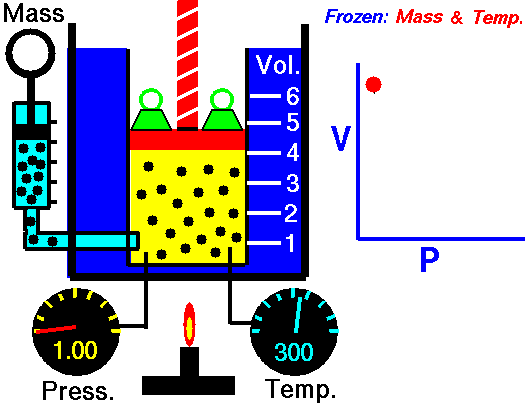# Boyle’s LawAir is a gas which has various properties which we can observe with our senses, including the gas pressure (p), temperature, mass, and the volume (V) which contains the gas. Careful, scientific observation has determined that these variables are related to one another, and the values of these properties determine the state of the gas.

In the mid 1600’s, Robert Boyle studied the relationship between the pressure and the volume of a confined gas held at a constant temperature. Boyle observed that the product of the pressure and volume are observed to be nearly constant. The product of pressure and volume is exactly a constant for an ideal gas:

$$\LARGE pV=\text{constant}$$

This relationship between pressure and volume is called Boyle’s Law in his honor. For example, suppose we have a theoretical gas confined in a jar with a piston at the top. The initial state of the gas has a volume equal to 4.0 cubic meters and the pressure is 1.0 kilopascal. With the temperature and number of moles held constant, weights are slowly added to the top of the piston to increase the pressure. When the pressure is 1.33 kilopascals the volume decreases to 3.0 cubic meters. The product of pressure and volume remains a constant (4 x 1.0 = 3 x 1.33333 ).

Provide feedback